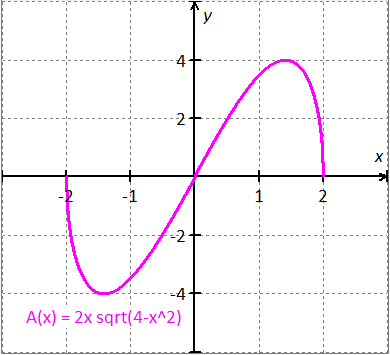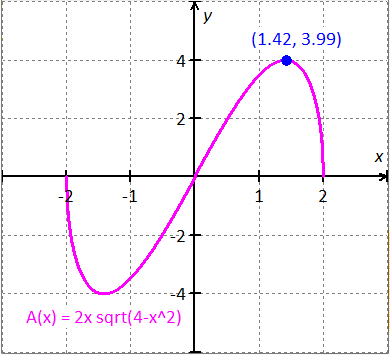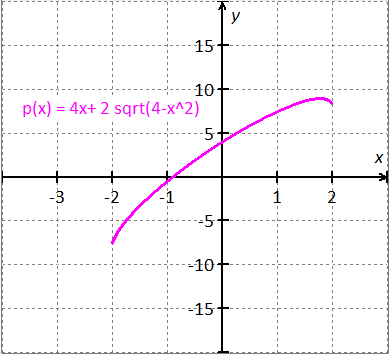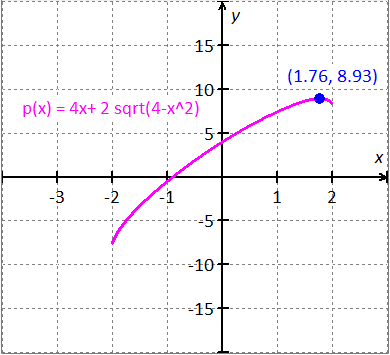PRE-ALGEBRA
ALGEBRA 1
GEOMETRY
ALGEBRA 2
TRIGONOMETRY
PRE-CALCULUS
CALCULUSPre-Calculus, 9th editionPAGE: 107 SET: Exercises PROBLEM: 8
Please look in your text book for this problem Statement(a) Express the areaof the rectangle as a function of.

Since the pointis located on the circle, the length of the rectangle is areand width of the rectangle is.

Formula for the area of the rectangle is, whereis the length andis the width of the rectangle.Substitutein..

(b) Express the perimeterof the rectangle as a function of.

The length of the rectangle is areand width of the rectangle is.

Formula for the perimeter of the rectangle is..

(c) Graph the function.

Graph of the function:Locate the maximum point on the graph.Observe the graph,is largest when.

(d) Graph the function.

Graph of the function:Locate the maximum point on the graph.Observe the graph,is largest when.

(a) The function is(b) The function is.

(c) Graph of the function:is largest when.

(d) Graph of the function:is largest when.TESTIMONIALS
 "I want to tell you that our students did well on the math exam and showed a marked improvement that, in my estimation, reflected the professional development the faculty received from you. THANK YOU!!!" June Barnett "Your site is amazing! It helped me get through Algebra." Charles "My daughter uses it to supplement her Algebra 1 school work. She finds it very helpful." Dan Pease
 Tweets by @mathskeydotcom

QUESTIONS? LET US HELP.
mathskey.com is not affiliated with any Publisher, Book cover, Title, Author names appear for reference only